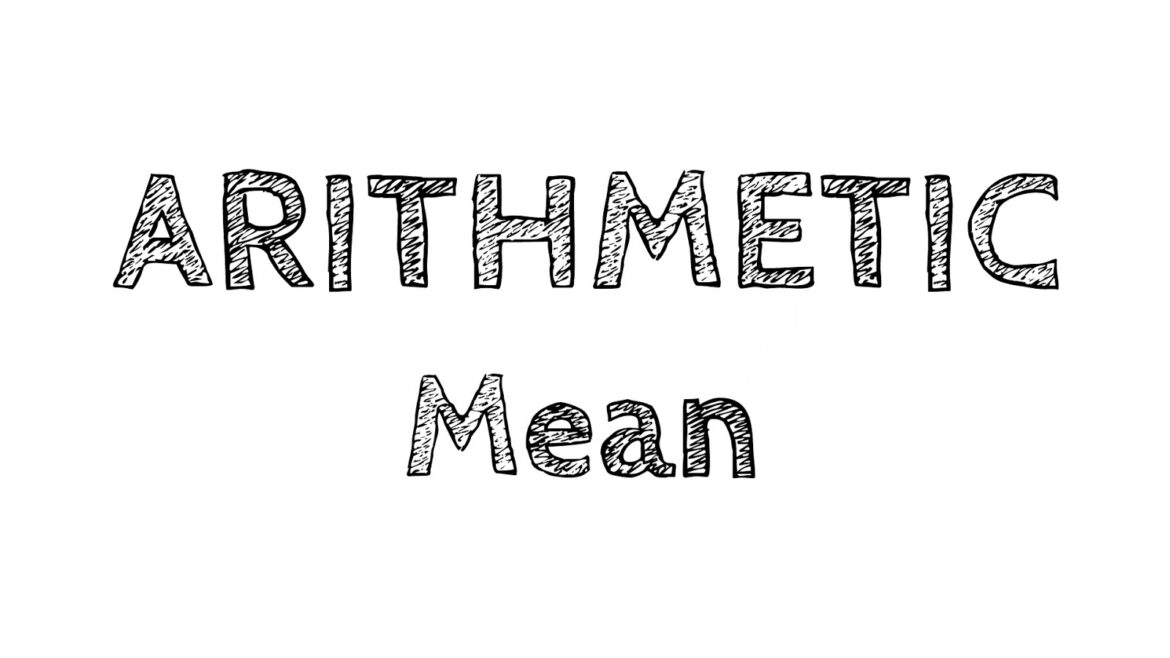# ARITHMETIC MEAN- MEANING, FORMULA, PROPERTIES

SELECT THE WORDS & LEVEL

Arithmetic mean is simply the average of the values given in the set of observations. It is so simple to calculate that even a normal human being, having little mathematical knowledge can easily calculate it. Having a rigid formula doesn’t change the calculated result. Let’s go through the basics of the arithmetic mean.

## What is an arithmetic mean?

• Arithmetic Mean in Statistics:

In central tendency, arithmetic mean is one of the most used tools. Also called as mean or average, it is the ratio of the sum of all the observations given in the set of data to the total number of observations in the data set. In statistics, the formula to calculate arithmetic mean depends on the amount and distribution of values in the data set.

• Arithmetic Mean in mathematics:

In mathematics, arithmetic is simply called arithmetic average or calculated central value of the given data set. To calculate the arithmetic mean, the calculated sum of all observations in the data set is divided by the total number of observations within that data set. The arithmetic average for equally distributed numbers in the data set is usually the middlemost number. Some of the examples of arithmetic mean are- the average weight of students in a class, average rainfall of an area, the average expenditure of employees in a firm, etc. The arithmetic mean of classified into two types:

• Simple Arithmetic Mean
• Weighted Arithmetic Mean

## What is the basic formula to calculate the arithmetic mean?

The formulas used to calculate the arithmetic mean depends on the amount and distribution of given data. But usually, the average value of a given set is calculated by dividing the sum of all observations in the data set by the total number of observations. The mathematical formula to calculate the arithmetic mean of a mentioned set of data is,

Arithmetic Mean = Sum of values in given data set / Total number of values in the given data set and, the symbolic representation of this formula is:

x̄ = ∑x/n

where,

x̄ = calculated arithmetic mean

∑ = values that are to be added

x = all values in the given data set

n = total number of values in the given data set

## What does the properties of arithmetic mean?

1. When all the values of a given set of data are the same, say ‘d’ then the calculated arithmetic mean is also ‘d’. For example, the set of data has 7 observations: 10,10,10,10,10,10,10.

So, the total of observations = 10 + 10 + 10 + 10 + 10 + 10 + 10 =70

Total number of observations = 7

Arithmetic mean = 70/7 = 10

1. When each value in the given data set is increased or decreased by a fixed value, then the value of the arithmetic mean also increases or decreases by the same number.
2. When each value in the given set is multiplied or divided by a fixed value, then the value of the arithmetic mean also gets multiplied or divided by the same number. Keep in mind that the values should be divided by a non-zero number as when the number is divided by zero then the calculated value can’t be defined.

Final Thoughts:

Cuemath, founded in 2013, is a platform that provides online classes to students so that they can easily understand the different concepts of math from the comfort of their homes. There are several classes available for arithmetic and geometric mean on this platform. In case you are facing any difficulty in solving the mathematical problems related to these topics then you can attend these classes at an affordable price.

Share